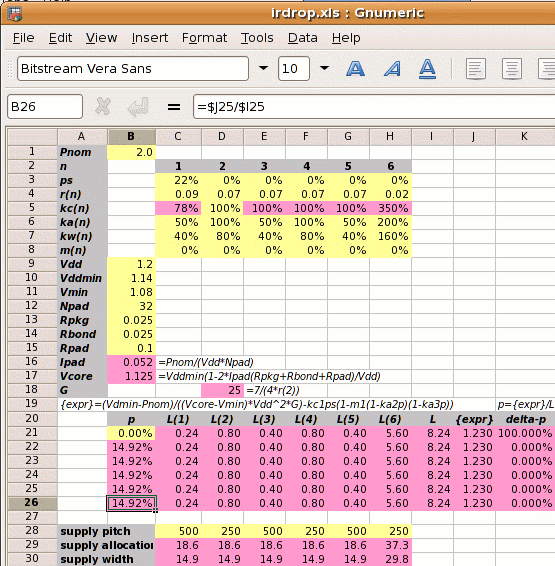Horizontal power straps are half the width (or twice the pitch) of vertical ones; metal resistivities are different; core power consumption is 2W with 32 core Vdd and 32 core Vss pads.

Step 1: Calculate Ipad and Vcore:

2⁄(1.2×32) = 0.052A
Vcore =
1.14×(1−2×0.052×(0.025+0.025+0.1)⁄1.2
1.125V

Step 2: Calculate the reference power supply conductance G:

G =
 7 4×r2

7 ⁄ (4 × 0.07) =  25 mhos

Step 3 is to set out the values of kan, kwn, kcn and mn for each metal layer, and use these to calculate the value of L.

metal layer 1 2 3 4 5 6
kan  50% 100%  50% 100%  50% 200%
power metal allocated coefficient
kwn  80%  80%  80%  80%  80%  80%
power metal used coefficient
kcn  78%¹ 100% 100% 100% 100% 350%²
conductivity coefficient
mn   0%   0%   0%   0%   0%   0%
core area blocked
¹78%=.07/.09; ²350%=.07/.02

Normally we can't calculate L directly because its value depends on p which we don't know. But in this case with m1‑6=0 we can

 L = kw1kc1(1-ps)(1-m1(1-ka2p)(1-ka3p))+ kw2kc2(1-m2(1-ka2p)(1-ka3p))+ kw3kc3(1-m3(1-ka2p)(1-ka3p))+ kw4kc4(1-m4(1-ka2p)(1-ka3p))+ kw5kc5(1-m5(1-ka2p)(1-ka3p))+ kw6kc6(1-m6(1-ka2p)(1-ka3p)) = ( 0.24 + 0.8 + 0.4 + 0.8 + 0.4 + 5.6 ) = 8.24

Step 4: Calculate the power strap allocation percentage p:

p =
 { Vddmin×Pnom −kc1×ps } × 1 (Vcore−Vmin)×Vdd2×G L
 { 1.14×2 −0.78×0.22 } × 1 (1.125−1.08)×1.22×25 8.24
(1.403−0.173)×0.121 = 14.92%

As shown on the right, a spreadsheet can be used to iterate to the answer.

If the designer sets the power strap pitch, then the supply allocation for each metal layer n is pitch×kan×p⁄2 and the supply width is pitch×kwn×p⁄2. An example is shown in the spreadsheet on the right where we have chosen a vertical power strap pitch of 250µm.

Step 5: Calculate the new core size. If the initial core size estimate without power straps is x, then with power straps the core size becomes x

 x′ = x = x = x = x+12.70% √(((1−ka2p)(1−ka3p)) √(0.8508×0.9254) 0.8873

The value 12.7% is called the IR Drop Adder.

This represents a more "normal" power busing scenario (but not necessarily a better one), where vertical power straps are preferred over horizontal ones. The extra use of metal-6 for power straps makes sense (a) because it is a long way from the transistors and so the impact on routing density should be less; and (b) because it is a thick metal layer with better conductivity.

Note the value of L=8.24 of which 5.6 comes from metal-6.

Design Attribute Value
Pnom core power consumption 2W
ps fraction of metal-1 in the standard cells used for power supplies 22% (for vsclib)
r1 resistivity of metal layer 1 in ohms per square 0.09Ω per sq.
r2-5 resistivity of metal layers 2-5 in ohms per square 0.07Ω per sq.
r6 resistivity of metal layer 6 in ohms per square 0.02Ω per sq.
ka1,3,5
 user defined   ratio of metal layers 1,3,5 allocated to power metal-2 allocated to power
50%
ka4
 user defined   ratio of metal layer 4 allocated to power metal-2 allocated to power
100%
ka6
 user defined   ratio of metal layer 6 allocated to power metal-2 allocated to power
200%
mn percentage of metal layer n blocked to power straps 0%
Vdd the nominal supply voltage 1.2V
Vddmin the minimum supply voltage, 5% less than nominal 1.14V
Vmin the desired voltage at the centre of the die, 10% less than the nominal 1.08V
Rpkg the resistance of the package leadframe 25mΩ
Rbond the resistance of the bond wire 25mΩ